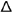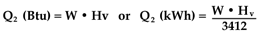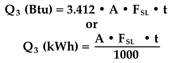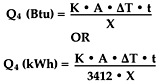INDUSTRIAL PROCESS INSTRUMENTS AND CONTROLS

## Power Estimating Equations

These equations are commonly used to estimate power requirements.

To obtain, for use with these equations, specific heat, latent heat of fusion and latent heat of vaporization of common substances, refer to the properties of non-metallic solids, metals, metals in a liquid state, liquids, and air and gases under Reference Data.Equation 1-- Heat Required To Raise The Temperature of A MaterialWhere: Q1 = Heat required to raise temperature W = Pounds of material CP = Specific heat of material (Btu/lb-ºF)T = Temperature rise of material          (TFinal - TInitial) ºF   Equation 2--Heat Required To Vaporize A Material (To Melt a Substance, Substitute Latent Heat of Fusion ---Hf---for Latent Heat of Vaporization)Where: Q2 = Heat required to vaporize W = Pounds of material Hv = Latent heat of vaporization (Btu/lb)   Equation 3--Heat Losses From SurfacesWhere: Q3 = Surface heat losses A = Surface area (ft2) FSL = Surface loss factors (W/ft2) evaluated at surface temperature t = Exposure time (hours)   Equation 4--Heat Losses By Conduction Through MaterialsWhere: Q4 = Conduction heat losses K = Thermal Conductivity (Btu · in/ft2 · ºF · hour) A = Heat transfer surface area (ft2) X = Thickness of material (inches) T = Temperature difference across material (T2 - T1)ºF t = Conduction time (hours)
 Go Shopping Search Products Basket Contents Track Order Company Information Terms & Conditions Your Privacy Manufacturers Contact Us! Technical Library Chemical Compatibility Cleanroom Standards Light Color Codes Conversion Charts Electrical Safety Electrical Symbols FCC Rules Heater Selection Humidity Motors Gears, Drives Ohm's Law Particle Contamination Power Equations Pressure Help Quick Formulas Research Links Temperature Conv t/c  Reference RTD Reference Time Standards 3-Phase Equations LonWorks Carbon Dioxide

SEARCH  INVENTORY